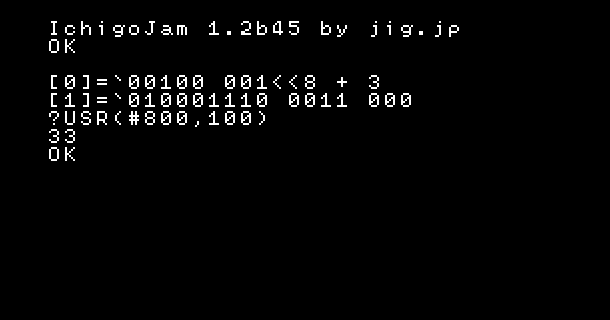About the machine language on IchigoJam, you cant't find how to divide (除算/割り算@ja) on the Arm Cortex-M0 command table. It's right. Arm Cortex-M0 has no commands of division. Instead you can use the function to divide.asm: R1=3 → 00100 001 [num]
asm: GOTO R3 → 010001110 [R3] 000
BASIC: PRINT 100 / 3 → 33

If you want to use the number as remainder (R1), call it!

=`00100 001<<8 + 3 =`1011010 100000000 =`010001111 0011 000 =`0100011000 001 000 =`1011110 100000000 ?USR(#800,100) 1

asm: R1=3 → 00100 001 [num]
asm: PUSH {LR} → 1011010 100000000
asm: GOSUB R3 → 010001111 [R3] 000
asm: R0=R1 → 0100011000 [R1] [R0]
asm: POP {PC} →　1011110 100000000
BASIC: PRINT 100 % 3 → 1

Enjoy programming with the simple machine language!